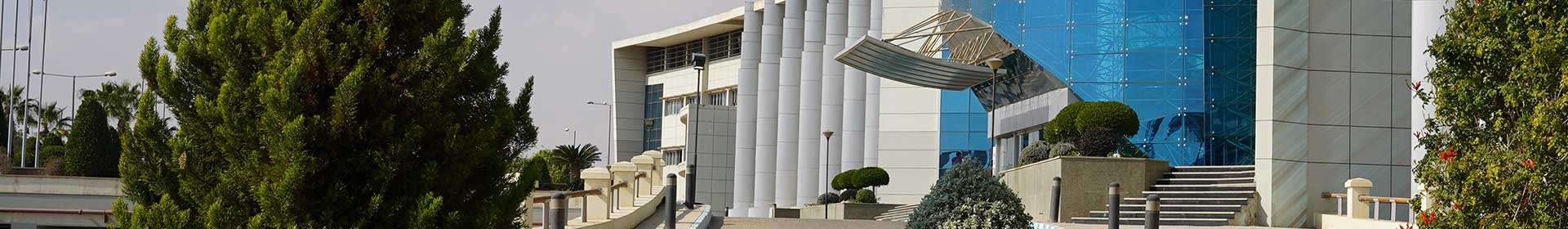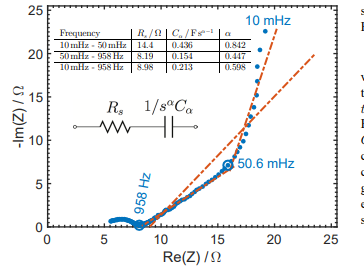Journal

# Further experimental evidence of the fractional-order energy equation in supercapacitors

By
Elwakil A.S.
Allagui A.
Freeborn T.J.
Maundy B.J.

Due to the dispersive porous nature of its material, carbon–carbon supercapacitors have a current–voltage relationship which is modeled by a fractional-order differential equation of the form i(t)=Cα[Formula presented] where α≤1 is a dispersion coefficient and Cα is a pseudo-capacitance not measurable in Farads. Hence, the energy stored in a capacitor, known to equal CV2/2 where C is the capacitance in Farad and V is the voltage applied, does not apply to a supercapacitor. In a recent work (Allagui et al., 2016), a fractional-order energy equation that enables the quantification of the energy stored in a supercapacitor when it is charged by a linear voltage ramp was derived. In addition, an effective capacitance (in proper Farad units) obtained from the time-domain analysis of the supercapacitor model under this type of charging was also derived. While some experimental results were given in Allagui et al. (2016), here we provide more experimental evidence of the applicability of the fractional-order energy equation using two commercial devices from two different vendors. We also show the effect of fast charging versus slow charging on the amount of energy stored in these supercapacitors. © 2017 Elsevier GmbH